Latest Banking jobs   »

# Quantitative Aptitude Quiz For IBPS SO Prelims 2022- 23rd December

Directions (1 -5): The following questions are accompanied by two statements (I) and (II). You have to determine which statements(s) is/are sufficient/necessary to answer the questions.
(a) Statement (I) alone is sufficient to answer the question but statement (II) alone is not sufficient to answer the questions.
(b) Statement (II) alone is sufficient to answer the question but statement (I) alone is not sufficient to answer the question.
(c) Both the statements taken together are necessary to answer the questions, but neither of the statements alone is sufficient to answer the question.
(d) Either statement (I) or statement (II) by itself is sufficient to answer the question.
(e) Statements (I) and (II) taken together are not sufficient to answer the question.

Q1. A bag contains some dairy milk, five Kitkat and seven five-star chocolates. Find number of dairy milk in the bag.
I . If one chocolate is drawn from the bag at random, then probability of being it Kitkat is 5/16.
II . If one chocolate is drawn from bag at random, then probability of being it either dairy milk or five – star is 11/16.

Q2. A shopkeeper bought a table at the price of Rs ‘2X’. Find the profit of shopkeeper on selling table (In Rs.)
I . Shopkeeper sold table at 20% profit after allowing two successive discounts of 20% & 4% respectively and difference between marked price and cost price is Rs. 1620.
II . Cost price of a chair is 75% of cost price of that table and difference between their cost price is Rs. 720. Shopkeeper marks up table by 56.25% and allows a discount of 23.2% on it.

Q3. How many men and women are working on this task?
I. (x – 4) men work for first (x – 8) days, after that (x+2) women complete the remaining work in (x – 8) days.
II. Ratio between work did by men to work did by women is 2 : 3 and efficiency of a man and a woman is equal.

Q4. If smaller train added three more compartments of 15 m each, then find in what time both trains cross each other running in opposite direction
I . Length of longer train is 25% more than that of smaller train and speed of longer train & smaller train is 81 km/hr & 108 km/hr respectively.
II . Longer & smaller train cross each other in 36 sec running in the same direction.

Q5. In a river there are two steamers A and B, where A covers 30 km downstream and B covers 30 km upstream. find the speed of B in still water.
I. B takes 2 hours more than A in covering the given distance and their respective directions and sum of still water speed of both the steamers is 16 km/hr.
II. Speed of current is 1 km/hr.

Q6. Probability of choosing a tiger over all animals (Ostrich, Tiger and Jackals) is 7/16. Find probability of picking a jackal, if ratio between number of heads to number of legs in park is 2 : 7.
(a) Cannot be determined
(b) 2/7
(c) 5/16
(d) 3/16
(e) ¼

Q7. Two types of ornaments are prepared by using gold and copper in two different proportions. In first ornaments, 6-gram gold is mixed with 5 gram copper and in second ornament 5 gram gold is mixed with 3 gram copper. If total quantity of gold and copper is 122 gram and 90 grams respectively then how many ornaments are prepared of second type?(total gold and copper is used)
(a) 15
(b) 10
(c) 7
(d) 20
(e) 9

Q8. In an annual exam of five subject, a student score in each subject was in the proportion of 7 : 8 : 9 : 10 : 11. If maximum marks in each subject are 200 and he scored 60% of total marks of all subjects then in how many paper he scored more than 55% marks?
(a) 1
(b) 3
(c) 4
(d) 5
(e) None of these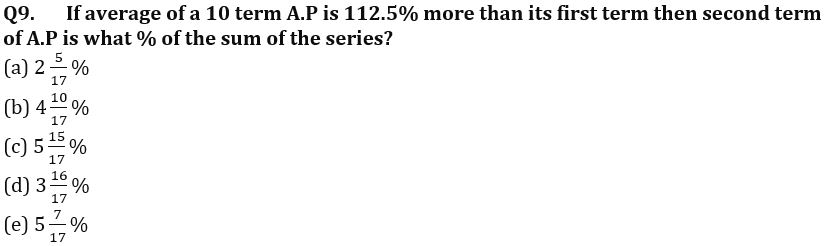Q10. Length of a rectangle is how much percent more than its breadth if area of rectangular plot is 480 sq. m. and when each side has been 5 m longer, the area would have been increased by 245 sq. m.?
(a) 50%
(b) 20%
(c) 10%
(d) 80%
(e) 40%

Directions (11-15): Read the given information carefully and answer the following questions.
A, B, C and D started a business in partnership. A invested Rs. 5000 for whole period whereas the average of initial investment for all four is Rs. 5975. Investment made by D is 25% more than B and ratio of investment made by B to that of C is 10 : 9. Time period for which B invested his amount is 2/3rd of that of A. Sum of time period for which C & D invested is equal to total time period for which A & B invested and profit share of C is 18/79th of total profit at the end of the year.

Q11. Find ratio of period of investment of C to that of D?
(a) 1 : 1
(b) 1 : 2
(c) 2 : 3
(d) 3 : 2
(e) 4 : 3

Q12. Difference between investment of D & C is what percent of difference between investment of B & D?
(a) 125%
(b) 100%
(c) 150%
(d) 140%
(e) 160%

Q13. If at the end of business term, total profit is Rs. 15,800, then find the difference between profit share of A & D.
(a) Rs. 800
(b) Rs. 1200
(c) Rs. 1000
(d) Rs. 1500
(e) None of these

Q14. Profit share of B is what percent of profit share of A?
(a) 80%
(b) 75%
(c) 60%
(d) 100%
(e) 65%

Q15. Investment of C & D together is approximately what percent of total investment of all the four?
(a) 45%
(b) 48%
(c) 50%
(d) 52%
(e) 54%

Solutions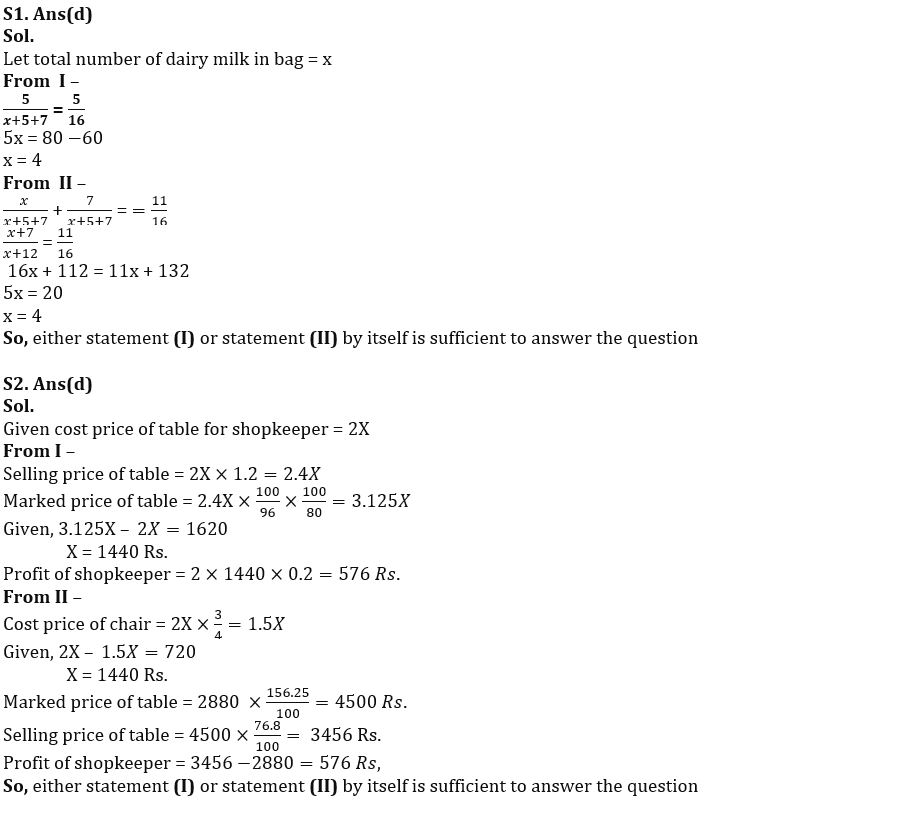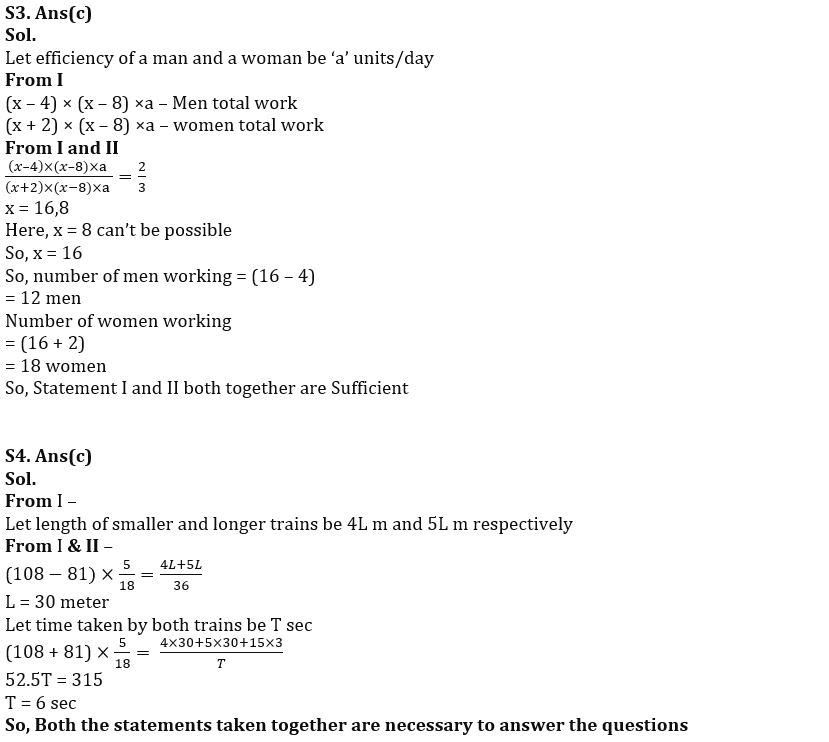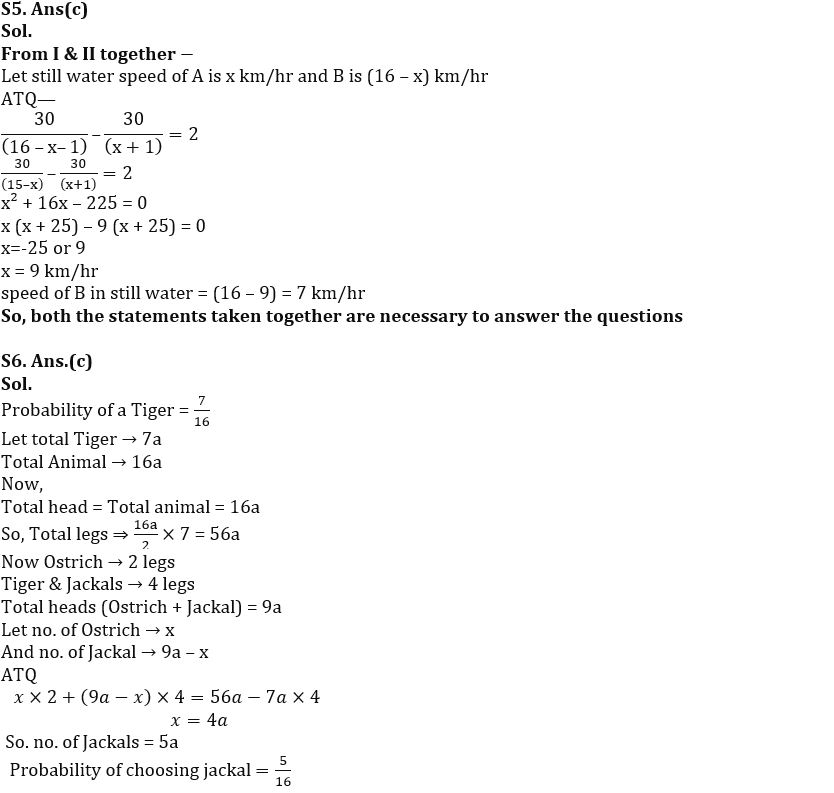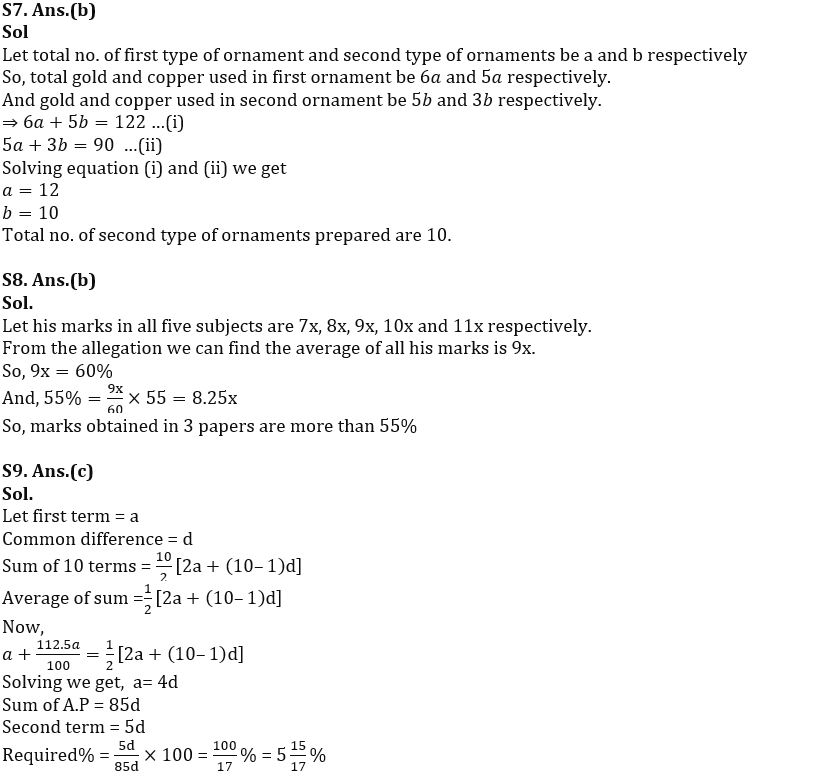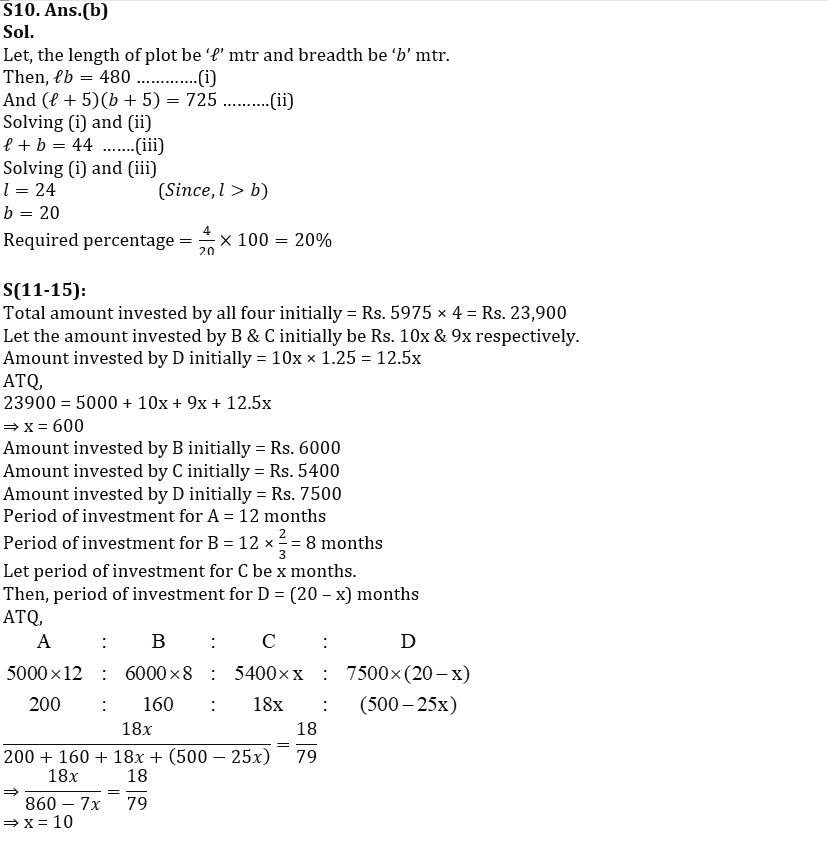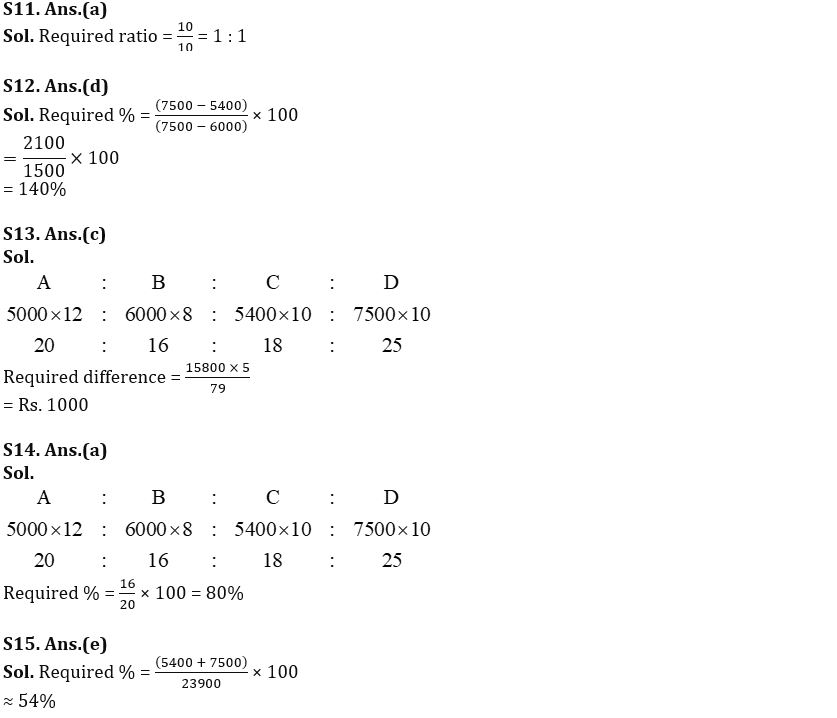#### Congratulations!Union Budget 2023-24: Free PDF